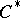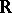The spaceof maximal ideals of the algebra of Bohr almost-periodic functions (cf. Banach algebra; Bohr almost-periodic functions). The Bohr almost-periodic functions form a commutative-algebraover the reals. The algebrais isometrically isomorphic to the algebraof all continuous functions on the compactum. The real lineis naturally imbedded inas an everywhere-dense subset (however, this imbedding is not a homeomorphism). The compactumhas the structure of a connected compact group which is identified with the group of characters of the real line if the latter is considered with the discrete topology. This isomorphism between the algebra of almost-periodic functions and the algebra of all continuous functions on the Bohr compactification makes it possible to simplify the proofs of a number of classical theorems. The concept of a Bohr compactification is also meaningful for the algebras of almost-periodic functions on other groups. In the case of the set of conditionally-periodic functions withindependent fixed periods, the-dimensional torus with these periods acts as the Bohr compactification.